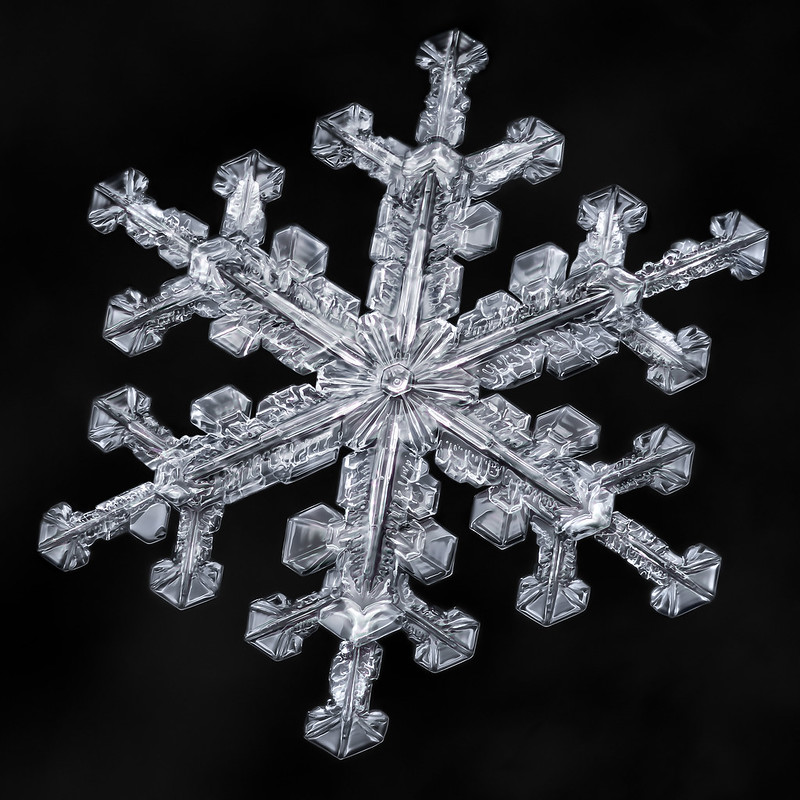### Wintersemester 2021/22

Dozent: Leonardo Patimo
Tutor: Vivien Vogelmann

## Content

A symmetry is a transformation that leaves an object unchanged. Knowing the symmetries of an object is often useful to determine many of its properties.Symmetries usually arise as actions of a group by automorphisms. Understanding the linear actions of a discrete group is equivalent to understanding the modules over the corresponding group ring. This naturally leads to considering more general noncommutative rings and to studying their structure.
The main topic in this course are the linear actions (or representations) of finite groups. We will first work in characteristic zero where representations are determined up to isomorphism by their characters. In particular, we will classify all the irreducible representations of the symmetric group.
We will then move to characteristic p>0, where the situation is more delicate, but through the notion of Brauer character we are able to recover some information about the representations of a finite group.
In the final part of the course ,if time permits, we will discuss linear actions of continuous group. We will see how these actions can be classified after rephrasing them in terms of the corresponding Lie algebras.

## Exercises

N.DatePDF
119.10Exercise Sheet 1
226.10Exercise Sheet 2
329.10Exercise Sheet 3
408.11Exercise Sheet 4
512.11Exercise Sheet 5
619.11Exercise Sheet 6
726.11Exercise Sheet 7
803.12Exercise Sheet 8
910.12Exercise Sheet 9
1013.01Exercise Sheet 10
1120.01Exercise Sheet 11
1227.01Exercise Sheet 12

## Lectures

S§ = Prof. Soergel's Skript, N§ = Supplementary Notes, T§ = Topolgie's Skript.

19.10. S§ 1.1 from Prof. Soergel's Skript: representations of groups
21.10. S§ 1.2-1.3: Group algebras; Jordan-Hölder theorem
26.10. S§ 1.4-1.5: Schur's Lemma, Semisimple Modules, S§ 2.1: Maschke's theorem for finite groups
28.10. S§ 1.8: Semisimple Rings and Artin-Wedderburn Theorem
29.10. Computation of the center of the group algebra, |irreducible representations| = |conjugacy classes|, S§ 2.2: Fourier Transform for Finite Groups. Some notes on the Discrete Fourier Transform.,
04.11. Substituted by Andreas Demleitner. N§ 5-6: Jacoboson Radical and Criteria for Semisimplicity. Andreas' notes.
09.11. S§ 2.3: Characters of representations. Orthogonality relations. Character table computed for S_3 and S_4.
11.11. S§ 2.4: Character and algebraic integers. Proof of Burnside's pq theorem (except 2.4.3 and 2.4.9.)
16.11. N§ 1.1-1.3: Tensor product of algebras and modules over a field. Simple modules over tensor product.
18.11. S§ 1.6: Jacobson's density theorem and Wedderburn's theorem. N§ 1.4 Tensor product of representations
23.11. S§ 3.1: Irreducible representations of the symmetric group in char 0 (first part: construction)
25.11. S§ 3.1: Irreducible representations of the symmetric group in char 0 (second part: proof that they cover all the classes). N§ 2: Tensor product over arbitrary ring
30.11. N§ 3 (up to 3.16): Induction and coinduction of representations. Induction and coinduction coincide for subgroup of finite index. Computation of the character of the induction.
02.12. N§ 3 (from 3.18), 4 (up to 4.5): Frobenius reciprocity. Weak and Strong form of Clifford theorem.
07.12. N§ 4 (from 4.6), 4.1-4.2: Clifford's correspondence. Applications of Clifford theory: subgroup of index 2 and p-groups
09.12. N§ 7-7.1: Krull-Schmidt theorem. Endomorphism rings of indecomposable modules are local.
14.12. N§ 8: Projective modules and idempotents.
16.12. N§ 9-10 (until Lemma 10.5): Radical of a module. Projective covers and essential morphisms
11.01. N§ 10 (from 10.6): Bijection between simple and indecomposable projectives. Decomposition of the regular module. S_3 in char 2
13.01. N§ 11: Restriction and induction of projectives. Projective covers compute multiplicities. Cartan matrix. A_4 in char 2
18.01. N§ 12: p-modular systems. From characteristic 0 to characteristic p. Brauer-Nesbitt's theorem and decomposition matrix
20.01. N§ 13: p-adic integers. Complete p-modular systems. Lifting idempotents and projective modules from characteristic p to characteristic 0.
25.01. N§ 14-15 (up to 15.5, without 14.1-14.2): Brauer reciprocity. Cartan matrix is symmetric. Linear independence of characters in char p
27.01. N§ 15 (from 15.6 to ): Brauer characters. Number of irreducible representations in char p
01.02. Topological groups. Representations of S^1 and Fourier series. Haar measure (without proof). Maschke theorem for compact groups. (see also T§ 4.1, 4.7.1-4.7.3)
03.02. Matrix coefficients. Orthogonality relations for matrix coefficients. Statement of Peter-Weyl theorem. (cf. T§ 4.2)
08.02. Peter-Weyl theorem: Sketch of the proof. Fourier transform for compact groups. (cf. T§ 4.5, 4.7.5) 10.02. Classification of the irreducible representations of SU(2,C) and SO(3,R). Decomposition of L^2(S^2) into SO(3,R)-representations.

## Supplementary Notes

This pdf contains some supplementary notes for the topics in the course not covered in the Skript, in particular Modular Representation Theory.

### Studien/Prüfungsleistungen

Die Studienleistung wird durch Bestehen der Klausur erbracht. Die Prüfungsleistung durch eine mündliche Prüfung.

The snowflake picture is "First Snowfall" by Don Komarechka released under CC0. You can have a look at other beautiful, and very symmetric!, snowflake pictures here.